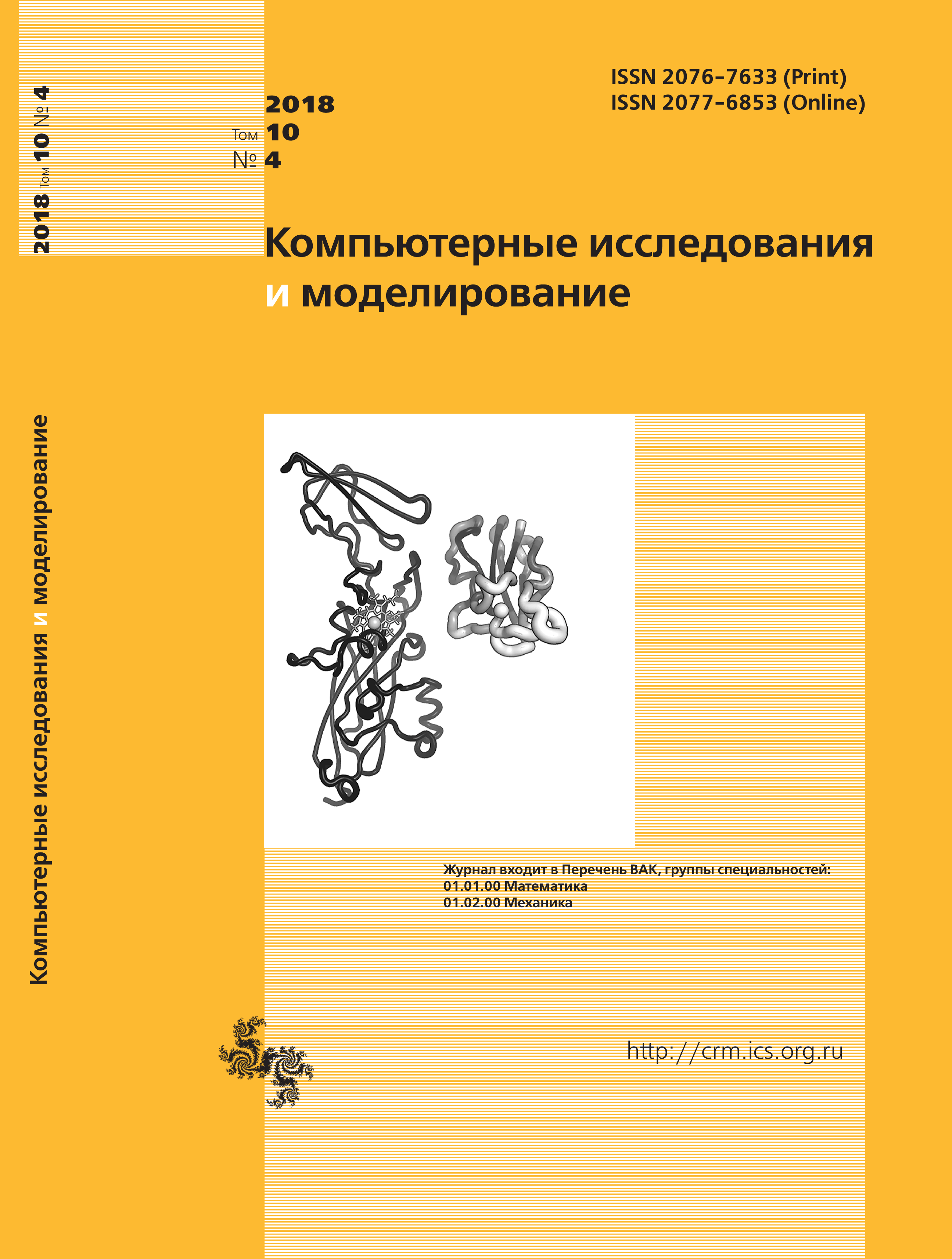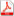Issue 4, 2018 Vol. 10

# All issues

High-Reynolds number calculations of turbulent heat transfer in FlowVision softwarepdf (10307K)  / List of references

This work presents the model of heat wall functions FlowVision (WFFV), which allows simulation of nonisothermal flows of fluid and gas near solid surfaces on relatively coarse grids with use of turbulence models. The work follows the research on the development of wall functions applicable in wide range of the values of quantity y+. Model WFFV assumes smooth profiles of the tangential component of velocity, turbulent viscosity, temperature, and turbulent heat conductivity near a solid surface. Possibility of using a simple algebraic model for calculation of variable turbulent Prandtl number is investigated in this study (the turbulent Prandtl number enters model WFFV as parameter). The results are satisfactory. The details of implementation of model WFFV in the FlowVision software are explained. In particular, the boundary condition for the energy equation used in high-Reynolds number calculations of non-isothermal flows is considered. The boundary condition is deduced for the energy equation written via thermodynamic enthalpy and via full enthalpy. The capability of the model is demonstrated on two test problems: flow of incompressible fluid past a plate and supersonic flow of gas past a plate (M = 3).

Analysis of literature shows that there exists essential ambiguity in experimental data and, as a consequence, in empirical correlations for the Stanton number (that being a dimensionless heat flux). The calculations suggest that the default values of the model parameters, automatically specified in the program, allow calculations of heat fluxes at extended solid surfaces with engineering accuracy. At the same time, it is obvious that one cannot invent universal wall functions. For this reason, the controls of model WFFV are made accessible from the FlowVision interface. When it is necessary, a user can tune the model for simulation of the required type of flow.

The proposed model of wall functions is compatible with all the turbulence models implemented in the FlowVision software: the algebraic model of Smagorinsky, the Spalart-Allmaras model, the SST $k-\omega$ model, the standard $k-\varepsilon$ model, the $k-\varepsilon$ model of Abe, Kondoh, Nagano, the quadratic $k-\varepsilon$ model, and $k-\varepsilon$ model FlowVision.

Keywords: turbulent boundary layer, high-Reynolds number calculations, wall functions, incompressible fluid, compressible gas, non-isothermal flow, heat flux, plate
Citation in English: Zhluktov S.V., Aksenov A.A., Savitskiy D.V. High-Reynolds number calculations of turbulent heat transfer in FlowVision software // Computer Research and Modeling, 2018, vol. 10, no. 4, pp. 461-481
Citation in English: Zhluktov S.V., Aksenov A.A., Savitskiy D.V. High-Reynolds number calculations of turbulent heat transfer in FlowVision software // Computer Research and Modeling, 2018, vol. 10, no. 4, pp. 461-481
DOI: 10.20537/2076-7633-2018-10-4-461-481

Full-text version of the journal is also available on the web site of the scientific electronic library eLIBRARY.RU

The journal is included in the Russian Science Citation Index

The journal is included in the List of Russian peer-reviewed journals publishing the main research results of PhD and doctoral dissertations.

International Interdisciplinary Conference "Mathematics. Computing. Education"

The journal is included in the RSCI

Indexed in Scopus